Drop a Query# Linear Regression in Julia

Linear Regression is a fundamental machine learning algorithm used to predict a numeric dependent variable based on one or more independent variables. The dependent variable (Y) should be continuous.

In this tutorial I explain how to build linear regression in Julia, with full-fledged post model-building diagnostics. To know more about the concepts behind linear regression, read: the complete introduction to linear regression.

## Content

1. Introduction to Linear Regression
2. Simple Linear Regression in Julia
3. Data Exploration
• Summary of the Data
• Outlier Analysis using Box Plot
• Distribution Analysis using Density Plot
• Correlation Analysis using Scatter Plot
4. Data Preprocessing
• One Hot Encoding
• Train-Test Split
5. Model Building
6. Model Diagnostics
• P value
• T Statistic
• R-Square
7. Model Predictions and Performance
• Mean Aboslute Error
• Mean Absolute Percentage Error
• Root Mean Square Error
• Mean Squared Error
• Error Distribution
8. Cross Validation
9. Multiple Linear Regression with Julia
10. Influencial Points using Cook’s Distance

## 1. Introduction to Linear Regression

Linear Regression is one of the most basic machine learning algorithms that is used to predict a dependent variable based on one or more independent variables. The dependent variable (Y) should be continuous. Linear regression finds the mathematical equation that best describes the Y variable as a function of the X variables (features). Once the equation is formed, it can be used to predict the value of Y when only the X is known.

This mathematical equation can be generalized as follows:

𝑌=𝛽1+𝛽2𝑋+𝜖

where 𝛽1 is the intercept and 𝛽2 is the slope. If there is only one X variable, it is called ‘Simple Linear Regression’. If more than one predictor (X) is involved, it is called ‘Multiple Linear Regression’. Nevertheless, the procedure the build either of them is pretty much the same.

Collectively, they are called regression coefficients and 𝜖 is the error term, the part of Y the regression model is unable to explain.

## 2. Simple Linear Regression with Julia

For this implementation, I would be using the Life Expectancy Data.

The goal is to predict the life expectancy of people in various countries depending on the various features and demographics. Let’s see how to do it in Julia.

### Import Packages and setup environment

Importing all the packages in the first step is always a good practice. So let’s load the packages here itself and enable printing max of 1000 columns in Jupyter cell.

# Import Packages
using Pkg  # Package to install new packages

# Install packages

using DataFrames
using CSV
using Plots
using Lathe
using GLM
using Statistics
using StatsPlots
using MLBase

# Enable printing of 1000 columns
ENV["COLUMNS"] = 1000


Let’s read the data using CSV.file function and later convert it to DataFrame object

# Read the file using CSV.File and convert it to DataFrame
df = DataFrame(CSV.File("../Data/Life_Expectancy_Data.csv"))
first(df,5)


## 3. Data Exploration

Before directly jumping to the model building, exploring the data is important. You should check what’s the size of the data frame. If it contains some missing values or outliers.

### Summary of the dataframe

println(size(df))
#> (1649, 22)

describe(df)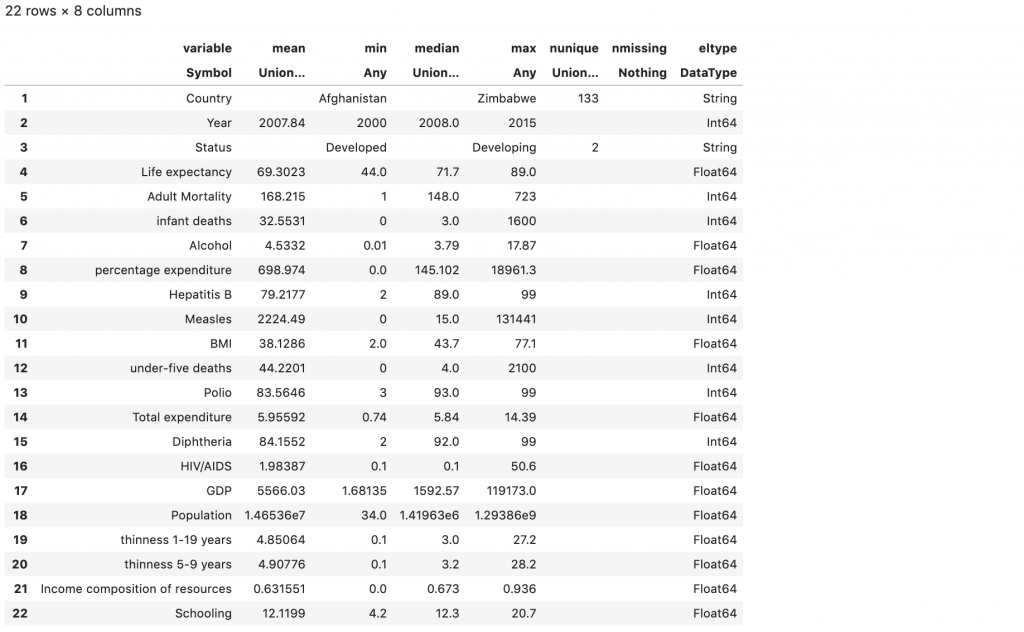By looking at the description, it looks like there are few spaces and special characters in the column names.

Let’s check the column names of the data frame. It’s a good practice to avoid spaces, special characters in the column names.

# Check column names
names(df)


#> 22-element Array{Symbol,1}:
#>  :Country
#>  :Year
#>  :Status
#>  Symbol("Life expectancy ")
#>  Symbol("infant deaths")
#>  :Alcohol
#>  Symbol("percentage expenditure")
#>  Symbol("Hepatitis B")
#>  Symbol("Measles ")
#>  Symbol(" BMI ")
#>  Symbol("under-five deaths ")
#>  :Polio
#>  Symbol("Total expenditure")
#>  Symbol("Diphtheria ")
#>  Symbol(" HIV/AIDS")
#>  :GDP
#>  :Population
#>  Symbol(" thinness  1-19 years")
#>  Symbol(" thinness 5-9 years")
#>  Symbol("Income composition of resources")
#>  :Schooling


Column names are having spaces and special characters. In Julia, if the column name has spaces, it is represented as a ‘Symbol’, else, it is prepended with a ‘:’, like you see in above snippet.

Let’s replace all these with under score _.

# Fix column names by replaceing ' ', '-', '/' with '_'
colnames = Symbol[]
for i in string.(names(df))
push!(colnames,Symbol(replace(replace(replace(strip(i)," " => "_"),"-" => "_"), "/" => "_")))
end

rename!(df, colnames);


### Outlier Analysis using Box Plot

Outlier is a data point or set of data points that differ significantly from other observations in the complete dataset.

Linear Regression works well when there aren’t any outliers present in the data. Let’s check out the outliers in y variable i.e. Life Expectancy

# Box Plot
boxplot(df.Life_expectancy, title = "Box Plot - Life Expectancy", ylabel = "Life Expectancy (years)", legend = false)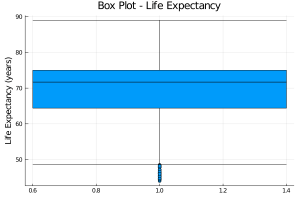There are few outliers in the data, represented by the points below the bottom leaf. Let’s remove these data points.

# Outlier removal
first_percentile = percentile(df.Life_expectancy, 25)
iqr_value = iqr(df.Life_expectancy)
df = df[df.Life_expectancy .>  (first_percentile - 1.5*iqr_value),:];


### Distribution Analysis using Density Plot

Linear Regression works well when the y variable is normally distributed or close to normal distribution. Let’s check out the distribution of y variable i.e. Life Expectancy

# Density Plot
density(df.Life_expectancy , title = "Density Plot - Life Expectancy", ylabel = "Frequency", xlabel = "Life Expectancy", legend = false)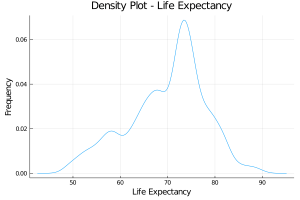The distribution does have a couple of mini peaks, which is indicative of a mixture of distributions. However, the overall distribution does have a bell curve. For the sake of understanding, let’s move to the next analysis

### Correlation Analysis using Scatter Plot

Linear Regression works well when the y variable is linearly correlated to the x variable. Let’s check out the correlation coefficient and scatter plot

# Correlation Analysis
println("Correlation of Life Expectancy with Adult Mortality Rate is ", cor(df.Adult_Mortality,df.Life_expectancy), "\n\n")

# Scatter plot
train_plot = scatter(df.Adult_Mortality,df.Life_expectancy, title = "Scatter Plot Life Expectancy vs Adult Mortality Rate", ylabel = "Life Expectancy", xlabel = "Adult Mortality Rate",legend = false)

#> Correlation of Life Expectancy with Adult Mortality Rate is -0.6756467502589402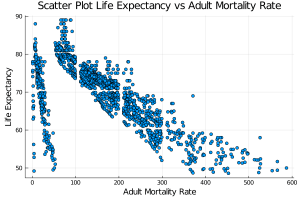The two features look linearly correlated. There are still a few chunk of points which are having different behaviour.

Some other feature might be able to explain this relation. You will see it while building the regression model with multiple features

## 4. Data Preprocessing

Data Preprocessing is one of the most important steps in model building. The data is already treated for outliers and column names. Let’s look at the categorical columns now.

### One Hot Encoding

One hot encoding is a process of converting categorical variables to form multiple numerical columns as there are categories. This is done so that, the variables could be fed into ML algorithms to do a better job in prediction.

2 categorical columns are present in the dataset. These columns need to be handled. Country column is having 133 unique values, so let’s drop it for this demonstration. Rather let’s encode the Status column.

# One hot encoding
scaled_feature = Lathe.preprocess.OneHotEncode(df,:Status)
select!(df, Not([:Status,:Country]))
first(df,5)


### Train-Test Split

Split the data to train and test set.

# Train test split
using Lathe.preprocess: TrainTestSplit
train, test = TrainTestSplit(df,.75)


## 5. Model Building

Built the linear regression model using “GLM” package. It’s very similar to the “GLM” package in R.

To train a linear regression model, use the lm() function that accepts a formula object as the first argument. Use the @formula to create the required formula object.

fm = @formula(Life_expectancy ~ Adult_Mortality)
linearRegressor = lm(fm, train)

StatsModels.TableRegressionModel{LinearModel{GLM.LmResp{Array{Float64,1}},GLM.DensePredChol{Float64,LinearAlgebra.Cholesky{Float64,Array{Float64,2}}}},Array{Float64,2}}

Coefficients:
───────────────────────────────────────────────────────────────────────────────────
Estimate  Std. Error   t value  Pr(>|t|)   Lower 95%   Upper 95%
───────────────────────────────────────────────────────────────────────────────────
(Intercept)      77.8244      0.301496   258.127     <1e-99  77.2329     78.4159
Adult_Mortality  -0.0493985   0.0015817  -31.2312    <1e-99  -0.0525018  -0.0462952
───────────────────────────────────────────────────────────────────────────────────


## 6. Model Dignostics

1. P Value
2. T Statistic
3. R Square

### P-value

A variable in linear regression model is said to be statistically significant only if the p-value is less than a pre-determined statistical significance level, which usually is 0.05.

In the above case only one feature is used to build the model. So there would be 2 p-values, one for the feature and one for the intercept. Both of these are less than 0.05, and hence statistically significant.

### T Statistic

The t value is the statistic metric calculated by dividing the beta coefficient (weight of the X variable) by its standard error. When I say, standard error, it is an estimate of the deviation of the beta coefficient.

A larger t-value indicates that it is less likely that the coefficient is not equal to zero purely by chance. So, the higher the t-value, the better.

### R Square

R-Square is a statistical measure which tells us the proportion of variation in the dependent (y) variable that is explained by different features (independent variables) in this model.

Higher the value of R-Square, the better. The max it can go is 1.

# R Square value of the model
r2(linearRegressor)
#> 0.4548557168286279


## 7 Model Prediction and Performance

1. Mean Aboslute Error
2. Mean Absolute Percentage Error
3. Root Mean Square Error
4. Mean Squared Error
5. Error Distribution

Let’s create the performance metrics. I would be looking at the performance metrics for both train data as well as test data.
Now that the model is ready, let’s predict on both the training and test data and cmopute squared errors.

# Prediction
ypredicted_test = predict(linearRegressor, test)
ypredicted_train = predict(linearRegressor, train)

# Test Performance DataFrame (compute squared error)
performance_testdf = DataFrame(y_actual = test[!,:Life_expectancy], y_predicted = ypredicted_test)
performance_testdf.error = performance_testdf[!,:y_actual] - performance_testdf[!,:y_predicted]
performance_testdf.error_sq = performance_testdf.error.*performance_testdf.error

# Train Performance DataFrame (compute squared error)
performance_traindf = DataFrame(y_actual = train[!,:Life_expectancy], y_predicted = ypredicted_train)
performance_traindf.error = performance_traindf[!,:y_actual] - performance_traindf[!,:y_predicted]
performance_traindf.error_sq = performance_traindf.error.*performance_traindf.error ;


Now, let’s calculate the Mean Absolute Percentage Error (MAPE).

# MAPE function defination
function mape(performance_df)
mape = mean(abs.(performance_df.error./performance_df.y_actual))
return mape
end


Now calculate Root Mean Squared Error (RMSE)

# RMSE function defination
function rmse(performance_df)
rmse = sqrt(mean(performance_df.error.*performance_df.error))
return rmse
end


The functions to compute errors is ready. Npow, let’s compute them on the test and training data.

# Test Error
println("Mean Absolute test error: ",mean(abs.(performance_testdf.error)), "\n")
println("Mean Aboslute Percentage test error: ",mape(performance_testdf), "\n")
println("Root mean square test error: ",rmse(performance_testdf), "\n")
println("Mean square test error: ",mean(performance_testdf.error_sq), "\n")


#> Mean Absolute test error: 4.239
#> Mean Absolute Percentage test error: 0.0609
#> Root mean square test error: 5.703
#> Mean square test error: 32.527

# Train  Error
println("Mean train error: ",mean(abs.(performance_traindf.error)), "\n")
println("Mean Absolute Percentage train error: ",mape(performance_traindf), "\n")
println("Root mean square train error: ",rmse(performance_traindf), "\n")
println("Mean square train error: ",mean(performance_traindf.error_sq), "\n")


#> Mean train error: 4.285
#> Mean Absolute Percentage train error: 0.0632
#> Root mean square train error: 5.811
#> Mean square train error: 33.773


Let’s see the residual error distribution. There’s shouldn’t be any pattern to the error and it should follow a normal distribution. I would be using a histogram for residual error analysis of both training as well as testing dataset

#### Error Distribution

# Histogram of error to see if it's normally distributed  on test dataset
histogram(performance_testdf.error, bins = 50, title = "Test Error Analysis", ylabel = "Frequency", xlabel = "Error",legend = false)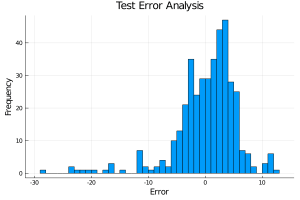# Histogram of error to see if it's normally distributed  on train dataset
histogram(performance_traindf.error, bins = 50, title = "Training Error Analysis", ylabel = "Frequency", xlabel = "Error",legend = false)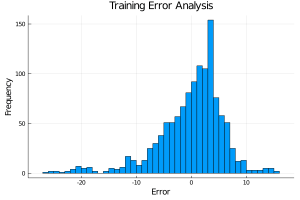It’s almost normally distributed, but still few outliers are there. Now let’s look at the actual and predicted values using a scatter plot. There shouldn’t be any specific pattern to it.

# Scatter plot of actual vs predicted values on test dataset
test_plot = scatter(performance_testdf[!,:y_actual],performance_testdf[!,:y_predicted], title = "Predicted value vs Actual value on Test Data", ylabel = "Predicted value", xlabel = "Actual value", legend = false)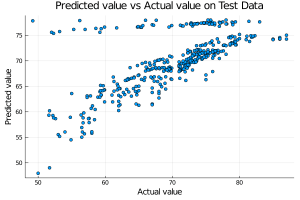# Scatter plot of actual vs predicted values on train dataset
train_plot = scatter(performance_traindf[!,:y_actual],performance_traindf[!,:y_predicted], title = "Predicted value vs Actual value on Train Data", ylabel = "Predicted value", xlabel = "Actual value",legend = false)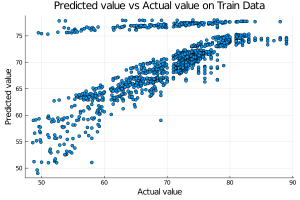There is definitely a problem with the model I just created, it’s not able to explain the effect on a few of the data points. I will explain this later on in the next section.

## 7. Cross Validation

Suppose, the model predicts satisfactorily on the 25% split (test data), is that enough to believe that your model will perform equally well all the time?

What do I mean by this?

A good model should perform equally well on new data (X vars) as it did on the training data. If the performance on new data (test data and any future data) deteriorates, it is an indication that the model may be overfit. That is, it is too complex that it explains the training data, but not general enough to perform as well on test.

So, it is important to rigorously cross validate the model’s performance as much as possible.

One way to do this rigorous testing, is to check if the model equation performs equally well when trained and tested on different mutually exclusive chunks of data.

That’s what cross validation is for. To understand cross validation in more detail, click here.
Let’s see the implementation.

# Cross Validation function defination
function cross_validation(train,k, fm = @formula(Life_expectancy ~ Adult_Mortality))
a = collect(Kfold(size(train), k))
for i in 1:k
row = a[i]
temp_train = train[row,:]
temp_test = train[setdiff(1:end, row),:]
linearRegressor = lm(fm, temp_train)
performance_testdf = DataFrame(y_actual = temp_test[!,:Life_expectancy], y_predicted = predict(linearRegressor, temp_test))
performance_testdf.error = performance_testdf[!,:y_actual] - performance_testdf[!,:y_predicted]

println("Mean error for set \$i is ",mean(abs.(performance_testdf.error)))
end
end

cross_validation(train,10)


#> Mean error for set 1 is 4.284476262508813
#> Mean error for set 2 is 4.452193180472949
#> Mean error for set 3 is 3.636780801512332
#> Mean error for set 4 is 4.2649906426451
#> Mean error for set 5 is 3.962627126108686
#> Mean error for set 6 is 4.125821731081508
#> Mean error for set 7 is 3.8671660294866927
#> Mean error for set 8 is 4.970669070855307
#> Mean error for set 9 is 4.626295912389546
#> Mean error for set 10 is 4.766206519686404


## 8. Multiple Linear Regression with Julia

By now, you should be having a better understanding of how to implement linear regression using only one independent variable in Julia. But in practical scenarios, there would be multiple independent variables. The core concepts I talked about would be the same. Let’s see the implementation.

I am using 7 features to build the regression model.

fm = @formula(Life_expectancy ~ Adult_Mortality + infant_deaths + Developing + BMI + Total_expenditure + HIV_AIDS   + Income_composition_of_resources)
linearRegressor = lm(fm, train)

StatsModels.TableRegressionModel{LinearModel{GLM.LmResp{Array{Float64,1}},GLM.DensePredChol{Float64,LinearAlgebra.Cholesky{Float64,Array{Float64,2}}}},Array{Float64,2}}

Life_expectancy ~ 1 + Adult_Mortality + infant_deaths + Developing + BMI + Total_expenditure + HIV_AIDS + Income_composition_of_resources

Coefficients:
───────────────────────────────────────────────────────────────────────────────────────────────────────
Estimate   Std. Error    t value  Pr(>|t|)   Lower 95%    Upper 95%
───────────────────────────────────────────────────────────────────────────────────────────────────────
(Intercept)                      61.9181      0.78997       78.3803     <1e-99  60.3681     63.468
Adult_Mortality                  -0.0213836   0.00133111   -16.0644     <1e-51  -0.0239952  -0.0187719
infant_deaths                    -0.00338122  0.000913086   -3.70307    0.0002  -0.0051727  -0.00158974
Developing                       -2.22109     0.361372      -6.14629    <1e-8   -2.93011    -1.51208
BMI                               0.0535088   0.00680987     7.85755    <1e-14   0.0401478   0.0668698
Total_expenditure                 0.160856    0.0521463      3.0847     0.0021   0.0585445   0.263167
HIV_AIDS                         -0.559147    0.0367949    -15.1963     <1e-46  -0.631339   -0.486955
Income_composition_of_resources  17.5357      0.784637      22.3488     <1e-91  15.9962     19.0752
───────────────────────────────────────────────────────────────────────────────────────────────────────

# R Square value of the model
r2(linearRegressor)


#> 0.7607534257484674


### Model Dignostics

The p-value, t value and R-Square value of all the features is statistically significant. So this is a good model from a diagnostics perspective. It’s better than the previous one. Let’s have a look at the performance of the model in prediction

### Model Prediction and Performance

# Prediction
ypredicted_test = predict(linearRegressor, test)
ypredicted_train = predict(linearRegressor, train)

# Test Performance DataFrame
performance_testdf = DataFrame(y_actual = test[!,:Life_expectancy], y_predicted = ypredicted_test)
performance_testdf.error = performance_testdf[!,:y_actual] - performance_testdf[!,:y_predicted]
performance_testdf.error_sq = performance_testdf.error.*performance_testdf.error

# Train Performance DataFrame
performance_traindf = DataFrame(y_actual = train[!,:Life_expectancy], y_predicted = ypredicted_train)
performance_traindf.error = performance_traindf[!,:y_actual] - performance_traindf[!,:y_predicted]
performance_traindf.error_sq = performance_traindf.error.*performance_traindf.error ;

# Test Error
println("Mean Absolute test error: ",mean(abs.(performance_testdf.error)), "\n")
println("Mean Aboslute Percentage test error: ",mape(performance_testdf), "\n")
println("Root mean square test error: ",rmse(performance_testdf), "\n")
println("Mean square test error: ",mean(performance_testdf.error_sq), "\n")


#> Mean Absolute test error: 2.743041547693274
#> Mean Absolute Percentage test error: 0.039794506972439955
#> Root mean square test error: 3.691776161739685
#> Mean square test error: 13.629211228389401

# Train  Error
println("Mean train error: ",mean(abs.(performance_traindf.error)), "\n")
println("Mean Aboslute Percentage train error: ",mape(performance_traindf), "\n")
println("Root mean square train error: ",rmse(performance_traindf), "\n")
println("Mean square train error: ",mean(performance_traindf.error_sq), "\n")


#> Mean train error: 2.8689694055517085
#> Mean Absolute Percentage train error: 0.04276809643988181
#> Root mean square train error: 3.8491608154153694
#> Mean square train error: 14.816038982929111


The error has been decreased as compared to the previous model.

Let’s see the residual error distribution.

### Error Distribution

# Histogram of error to see if it's normally distributed  on test dataset
histogram(performance_testdf.error, bins = 50, title = "Test Error Analysis", ylabel = "Frequency", xlabel = "Error",legend = false)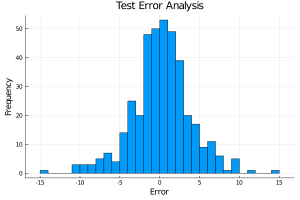# Histogram of error to see if it's normally distributed  on train dataset
histogram(performance_traindf.error, bins = 50, title = "Training Error Analysis", ylabel = "Frequency", xlabel = "Error",legend = false)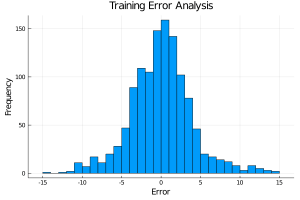It’s almost normally distributed, the error distribution is also better than the previous model. Let’s look at the predicted values using the scatter plot

# Scatter plot of actual vs predicted values on test dataset
test_plot = scatter(performance_testdf[!,:y_actual],performance_testdf[!,:y_predicted], title = "Predicted value vs Actual value on Test Data", ylabel = "Predicted value", xlabel = "Actual value", legend = false)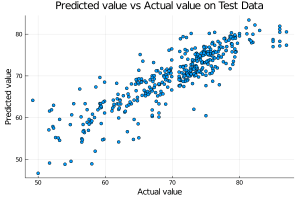# Scatter plot of actual vs predicted values on train dataset
train_plot = scatter(performance_traindf[!,:y_actual],performance_traindf[!,:y_predicted], title = "Predicted value vs Actual value on Train Data", ylabel = "Predicted value", xlabel = "Actual value",legend = false)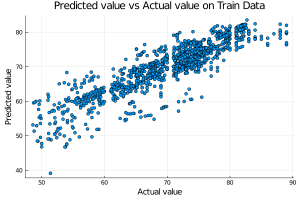The predictions are very close to the actual values. The pattern in a few data points has also been explained now by independent variables. Let’s have a look at the cross-validation score.

### Cross Validation

cross_validation(train,10, fm)


#> Mean error for set 1 is 2.8945332068834726
#> Mean error for set 2 is 2.559176516353831
#> Mean error for set 3 is 2.564319867198253
#> Mean error for set 4 is 2.441408656510297
#> Mean error for set 5 is 2.957111326596502
#> Mean error for set 6 is 3.0836969581692286
#> Mean error for set 7 is 3.034633016712589
#> Mean error for set 8 is 3.1742828015038684
#> Mean error for set 9 is 2.853670477762846
#> Mean error for set 10 is 3.5847443679028563


The error is not varying much. the model is not overfitted.

By looking at the different parameters and evaluation metrics, it can be concluded that the model is quite good.

Now let’s look at one of the very less knows but high-value topic in linear regression.

## 9. Influencial Points using Cook’s Distance

### Cook’s Distance

Cook’s distance is a measure computed with respect to a given regression model and therefore is impacted only by the X variables included in the model.

But, what does cook’s distance mean?

It computes the influence exerted by each data point (row) on the predicted outcome.

The cook’s distance for each observation i measures the change in Ŷ (fitted Y) for all observations with and without the presence of observation i, so we know how much the observation i impacted the fitted values. Mathematically, cook’s distance Di for observation i is computed as: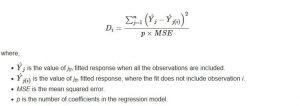# Cook's Distance function definition
function cook_distance(train,n_coeff = 2, fm = @formula(Life_expectancy ~ Adult_Mortality))
linearRegressor = lm(fm, train)

# MSE calculation
performance_traindf = DataFrame(y_actual = train[!,:Life_expectancy], y_predicted = predict(linearRegressor, train))
performance_traindf.error = performance_traindf[!,:y_actual] - performance_traindf[!,:y_predicted]
performance_traindf.error_sq = performance_traindf.error.*performance_traindf.error
mse = mean(performance_traindf.error_sq)

cooks_distance = []
for i in 1:size(train)
total_rows = collect(1:size(train))
training_rows = deleteat!(total_rows, total_rows .== i)
temp_train = train[training_rows,:]
linearRegressor = lm(fm, temp_train)

temp_performance_traindf = performance_traindf[training_rows,:]

# Predicted Value
ypredicted_train = predict(linearRegressor, temp_train)

# Test Performance DataFrame
performance_df = DataFrame(yi = temp_performance_traindf.y_predicted, yj = ypredicted_train)
performance_df.y_diff = performance_df[!,:yi] - performance_df[!,:yj]
performance_df.y_diff_sq = performance_df.y_diff.*performance_df.y_diff
cooks_d = sum(performance_df.y_diff_sq)/(n_coeff*mse)
push!(cooks_distance,cooks_d)
end
cooks_distance_df = DataFrame(index = collect(1:size(train)), cooks_d = cooks_distance )
return cooks_distance_df
end

# Calculate Cook's Distance
cooks_distance_df = cook_distance(train, 9, fm);
mean_cooks_distance = mean(cooks_distance_df.cooks_d)
influencial_points = cooks_distance_df[cooks_distance_df.cooks_d .> 4*mean_cooks_distance,:]
println(mean_cooks_distance)
#> 0.0012888653127413436


### Influence measures

In general use, those observations that have a cook’s distance greater than 4 times the mean may be classified as influential. This is not a hard boundary.

Let’s see these data points on the plot

plot = scatter(cooks_distance_df.index, cooks_distance_df.cooks_d, title = "Cook's Distance Analysis", ylabel = "Cook's Distance", xlabel = "Index",  label = ["Cook's Distance"], ylim = (0,0.03))
hline!([mean_cooks_distance], lw = 3,  label = ["Mean Cook's Distance"])
plot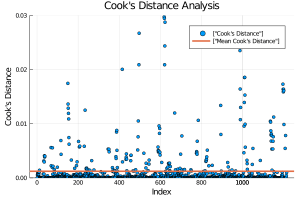Now let’s find out the influential rows from the original data. If you extract and examine each influential row 1-by-1 (from below output), you will be able to find reason out why that row turned out influential. It is likely that one of the X variables included in the model had extreme values.

high_cooksd_points = cooks_distance_df[cooks_distance_df.cooks_d .> 0.02,:]
influencial_points_df = train[high_cooksd_points.index,[:Adult_Mortality, :infant_deaths, :Developing, :BMI, :Total_expenditure, :HIV_AIDS,   :Income_composition_of_resources]]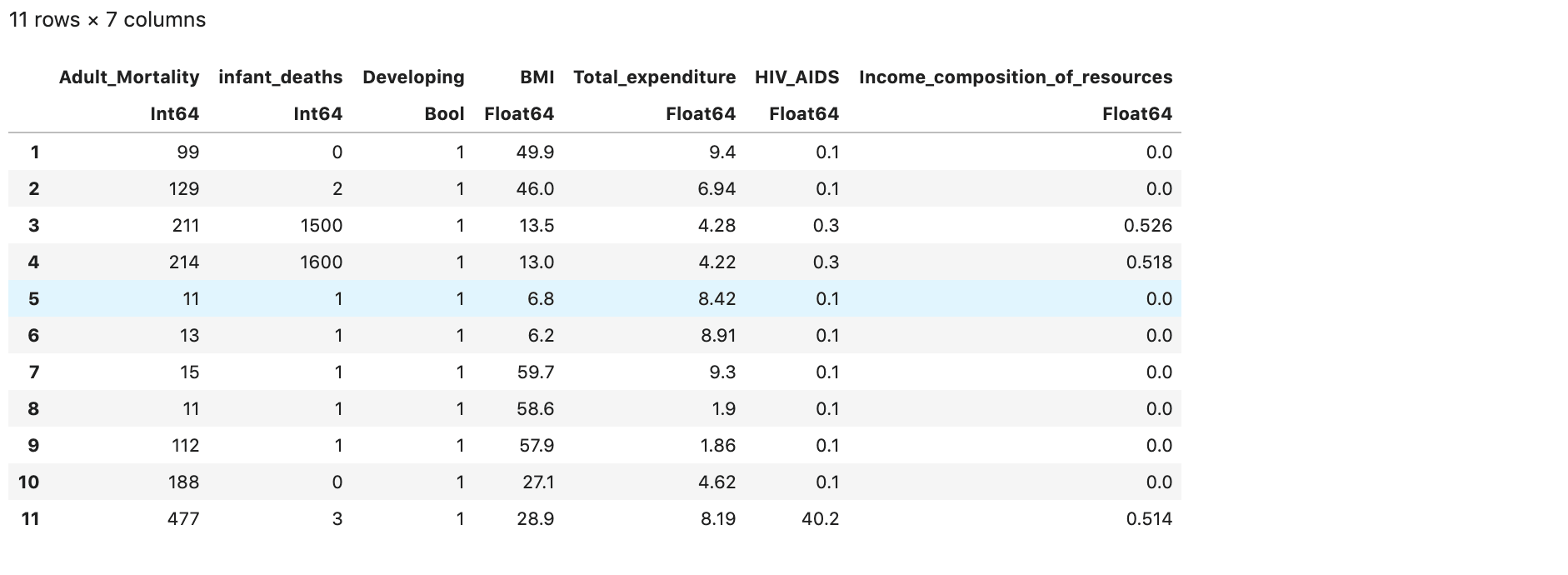• Row 8,9,10 are having very high value of adult mortality rate and HIV
• Row 1,3,4 are having very less BMI
• Row 5,6,7 are having very less Total Expenditure

## Conclusion

I have covered the basic concepts about linear regression and the implementation in Julia. Next, I will see you with more Data Science oriented topics in Julia.Course Preview

## Machine Learning A-Z™: Hands-On Python & R In Data Science

### Free Sample Videos:#### Machine Learning A-Z™: Hands-On Python & R In Data Science#### Machine Learning A-Z™: Hands-On Python & R In Data Science#### Machine Learning A-Z™: Hands-On Python & R In Data Science#### Machine Learning A-Z™: Hands-On Python & R In Data Science